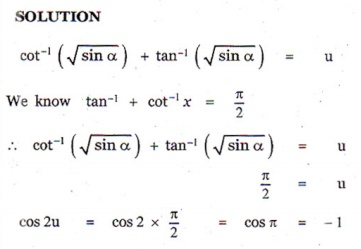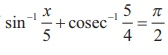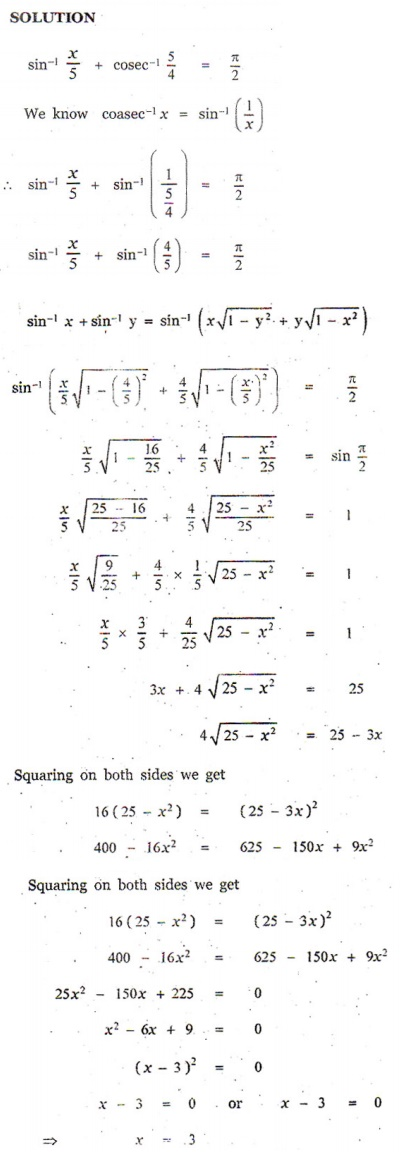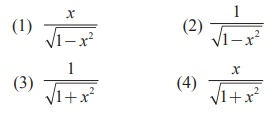Home | | Maths 12th Std | Choose the correct answers

## Chapter: 12th Mathematics : UNIT 4 : Inverse Trigonometric Functions

Choose the correct or the most suitable answer from the given four alternatives, Multiple choice questions with Answers, Solution - Maths Book back 1 mark questions and answers with solution for Exercise Problems

Choose the correct or the most suitable answer from the given four alternatives.

1. The value of sin(cos x), 0   π is

(1) π - x

(2) x  - π/2

(3) π/2 - x

(4) x - π2. If sin+ siny (2π/3) ; then cos+ cosis equal to

(1) 2π /3

(2) π /3

(3) π /6

(4) π3.is equal to

(1) 2π

(2) π

(3) 0

(4) tan-1 (12/65)4. If sin= 2 sinα has a solution, then

(1) |α| ≤ (1/√2)

(2) |α| ≥ (1/√2)

(3) |α| < (1/√2)

(4) |α| > (1/√2)5.is valid for

(1) − π ≤ x ≤ 0

(2) 0 ≤ x ≤ π

(3) -π/2 ≤ x ≤ π/2

(4) -π/4 ≤ x ≤ 3π/46. If sin-1 + sin-1 + sin-1= 3π / 2 , the value of x2017  + y2018 + z2019 – [ 9/ (x101 + y101 + z101)] is

(1) 0

(2) 1

(3) 2

(4) 3

7. If cot−1 =2π/3 for some  , the value of tan−1 is

(1) – π/10

(2) π/5

(3) π/10

(4) - π/58. The domain of the function defined by (x) = sin-1√(x-1) is

(1) [1,2]

(2) [-1,1]

(3) [0,1]

(4) [-1,0]9. If  =1/5 , the value of  cos (cos1  + 2 sin1  x) is

(1) – √(24/25)

(2) √(24/25)

(3) 1/5

(4) -1/510.is equal toAns: (4)11. If the function (x= sin(x2   3) , then  x  belongs to

(1) [-1,1]

(2) [√2,2]

(3) [-2-√2]U[√2,2]

(4) [-2,- √2]12. If cot2 and cot3 are two angles of a triangle, then the third angle is

(1) π/4

(2) 3π/4

(3) π/6

(4) π/313.. Then is a root of the equation

(1) x2 − − 6 = 0

(2) x2 − −12 = 0

(3) x2 + −12 = 0

(4) x2 + − 6 = 014. sin(2 cos1) + cos(1 2 sinx) =

(1) π/2

(2) π/3

(3) π/4

(4) π/615. Ifthen cos 2is equal to

(1) tan2 α

(2) 0

(3) -1

(4) tan 2α16. If |x| ≤ 1 , then 2tan-1 x - sin-1 2x/ (1+ x2) is equal to

(1) tan-1 x

(2) sin-1 x

(3) 0

(4) π17. The equation tan-1x - cot-1 x = tan-1 ( 1/√3) has

(1)  no solution

(2) unique solution

(3) two solutions

(4) infinite number of solutions18.If, then is equal to

(1) 1/2

(2) 1/√5

(3) 2/√5

(4) √3/219. Ifthen the value of x is

(1) 4

(2) 5

(3) 2

(4) 320. sin(tanx), | |<1 is equal toAns: (4)Choose the correct or the most suitable answer from the given four alternatives.

1. The value of sin(cos x), 0   π is

(1) π - x

(2) x  - π/2

(3) π/2 - x

(4) x - π

2. If sin+ siny (2π/3) ; then cos+ cosis equal to

(1) 2π /3

(2) π /3

(3) π /6

(4) π

3.is equal to

(1) 2π

(2) π

(3) 0

(4) tan-1 (12/65)

4. If sin= 2 sinα has a solution, then

(1) |α| ≤ (1/√2)

(2) |α| ≥ (1/√2)

(3) |α| < (1/√2)

(4) |α| > (1/√2)

5.is valid for

(1) − π ≤ x ≤ 0

(2) 0 ≤ x ≤ π

(3) -π/2 ≤ x ≤ π/2

(4) -π/4 ≤ x ≤ 3π/4

6. If sin-1 + sin-1 + sin-1= 3π / 2 , the value of x2017  + y2018 + z2019 – [ 9/ (x101 + y101 + z101)] is

(1) 0

(2) 1

(3) 2

(4) 3

7. If cot−1 =2π/3 for some  , the value of tan−1 is

(1) – π/10

(2) π/5

(3) π/10

(4) - π/5

8. The domain of the function defined by (x) = sin-1√(x-1) is

(1) [1,2]

(2) [-1,1]

(3) [0,1]

(4) [-1,0]

9. If  =1/5 , the value of  cos (cos1  + 2 sin1  x) is

(1) – √(24/25)

(2) √(24/25)

(3) 1/5

(4) -1/5

10.is equal toAns: (4)

11. If the function (x= sin(x2   3) , then  x  belongs to

(1) [-1,1]

(2) [√2,2]

(3) [-2-√2]U[√2,2]

(4) [-2,- √2]

12. If cot2 and cot3 are two angles of a triangle, then the third angle is

(1) π/4

(2) 3π/4

(3) π/6

(4) π/3

13.. Then is a root of the equation

(1) x2 − − 6 = 0

(2) x2 − −12 = 0

(3) x2 + −12 = 0

(4) x2 + − 6 = 0

14. sin(2 cos1) + cos(1 2 sinx) =

(1) π/2

(2) π/3

(3) π/4

(4) π/6

15. Ifthen cos 2is equal to

(1) tan2 α

(2) 0

(3) -1

(4) tan 2α

16. If |x| ≤ 1 , then 2tan-1 x - sin-1 2x/ (1+ x2) is equal to

(1) tan-1 x

(2) sin-1 x

(3) 0

(4) π

17. The equation tan-1x - cot-1 x = tan-1 ( 1/√3) has

(1)  no solution

(2) unique solution

(3) two solutions

(4) infinite number of solutions

18.If, then is equal to

(1) 1/2

(2) 1/√5

(3) 2/√5

(4) √3/2

19. Ifthen the value of x is

(1) 4

(2) 5

(3) 2

(4) 3

20. sin(tanx), | |<1 is equal toAns: (4)Tags : Inverse Trigonometric Functions , 12th Mathematics : UNIT 4 : Inverse Trigonometric Functions
Study Material, Lecturing Notes, Assignment, Reference, Wiki description explanation, brief detail
12th Mathematics : UNIT 4 : Inverse Trigonometric Functions : Choose the correct answers | Inverse Trigonometric Functions